# Chapter 15 Simulate for Probabilistic thinking

These notes accompany portions of Chapter 5 — Probability — of our textbook, which we also examine in the previous section. The reading below is required, Whitlock and Schluter (2020) is not.

Motivating scenarios: We want to build an intuitive feel for probability that we can build from simulation.

Learning goals: By the end of this chapter you should be able to

• Develop simulations to solidify our probabilistic intuition.

## 15.1 Why Simulate?

A lot of statistics is wrapping our heads around probabilistic thinking. To me the key here is to get good intuition for probabilistic thinking. Mathematical formulae (like those introduced last chapter) are essential for rapid computation and help some people understand the concepts. But I personally understand things better when I see them, so I often simulate.

I simulate to:

1. Make sure my math is right.
2. Build intuition for probability problems.
3. Solve probability questions that do not have a mathematical solution.

So, how do we simulate? Last Chapter we introduced some simulation that was built into the seeing theory app, but that app will not store our output or keep a record of our code.

More naturally, we can code probabilistic events. We can use a bunch of R functions to simulate, depending on our goals. Today, we’ll the sample() function, which we saw when we considering sampling and uncertainty in our estimates.

Our goal is to use simulation to reinforce concepts in probability and to develop an intuition for probabilistic outcomes. This should solidify our mathematical understanding of the rules of probability developed in the previous chapter.

## 15.2 Simulating exclusive events with the sample() function in R

While I love the seeing theory app, it is both limiting and does not store our outoput or keep a record of our code. Rather we use the sample() function in R.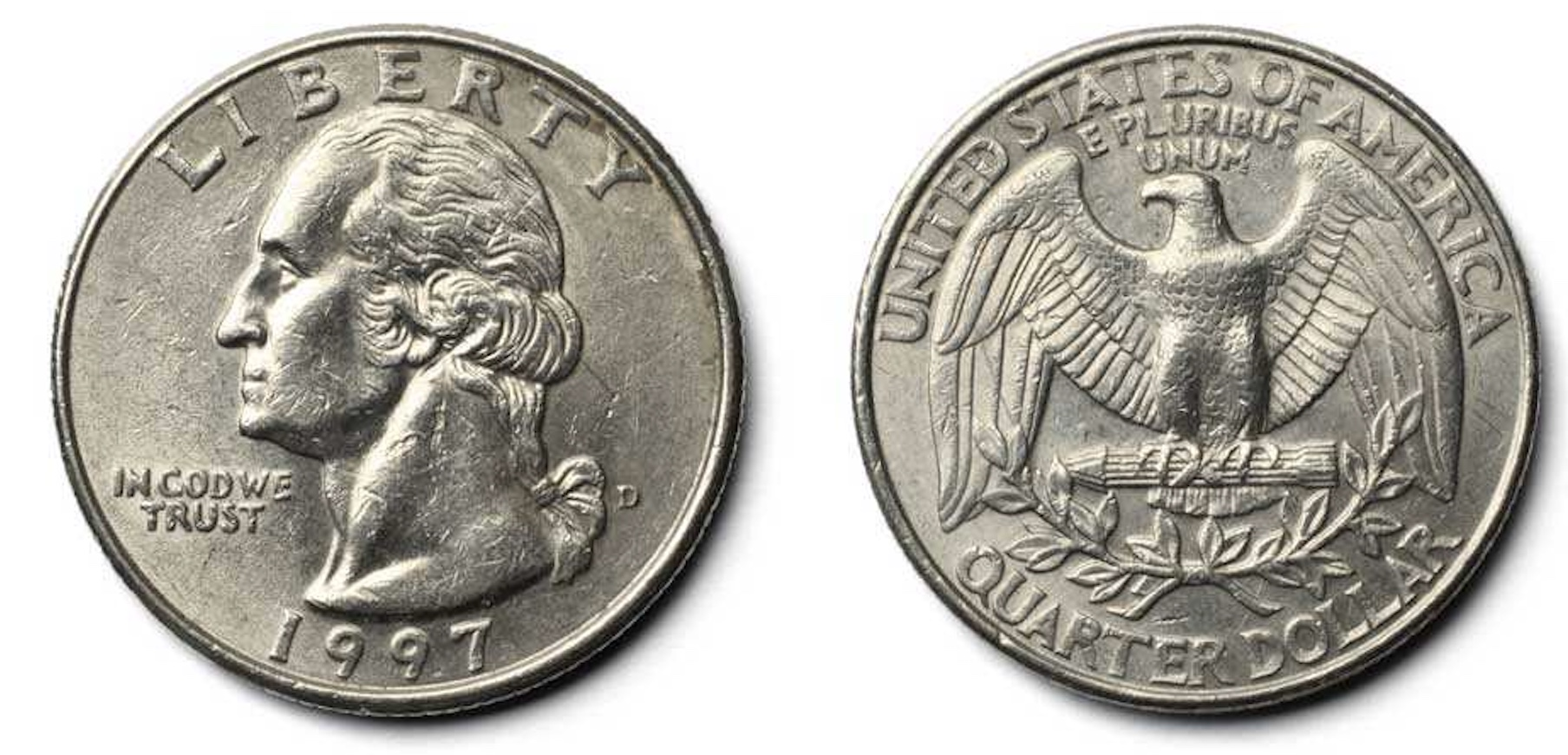Let’s simulate the simple our coin-tossing example introduced in the previous chapter to get started. Remember that of sample space for a single coin flip is

• Coin lands heads side up
• Coin lands tails side up

To flip a single coin with a 40% chance of landing heads up, we type:

sample(x = c("Heads","Tails"), size = 1, replace = TRUE, prob = c(.4,.6))
##  "Tails"

To flip a sample of five coins, each with a 45% chance of landing tails up, we type:

sample(x = c("Heads","Tails"), size = 5, replace = TRUE, prob = c(.55,.45))
##  "Heads" "Heads" "Heads" "Tails" "Tails"

If we do not give R a value for prob (e.g. x = c("Heads","Tails"), size = 5, replace = TRUE)) it assumes that each outcome is equally probable.

### Keeping track of and visualizing simulated proportions from a single sample in R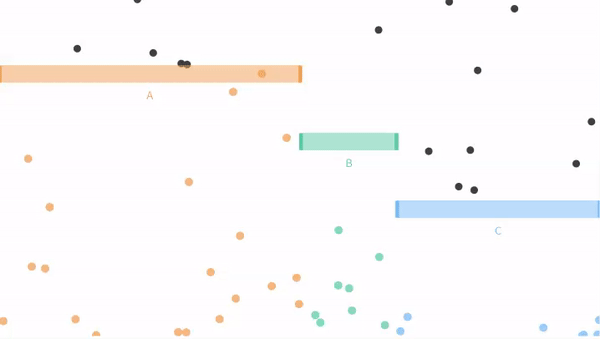Now let us move on from our coin flipping example to our ball dropping examples from seeing theory. Remember that potential outcomes are

• The ball can fall through the orange bin (A), with probability P(A) = 3/6.
• The ball can fall through the green bin (B), with probability P(B) = 1/6.
• The ball can fall through the blue bin (C) with probability P(C) = 2/6.

We can set these probabilities in R by assigning each to a variable, as follows

P_A      <- 3/6  # aka 1/2
P_B      <- 1/6  # aka 1/6
P_C      <- 2/6  # aka 1/3

We can then write a signle line of R code, to select ten balls with replacement

sample(c("A", "B", "C"), size = 10, replace = TRUE, prob = c(P_A, P_B, P_C))
##   "A" "A" "B" "A" "A" "C" "C" "A" "C" "A"

The code above produces a vector, but we most often want data in tibbles. Let’s do this, but for funzies, lets make a sample of five hundred balls, with probabilities equal to those in Figure above. To do so, we simply make a tibble and assign our vector to a column

n_balls  <- 500
ball_dist_exclusive <- tibble(balls = sample(x = c("A","B","C"),
size  = n_balls,
replace = TRUE,
prob = c(P_A, P_B, P_C)))

Let’s have a look at the result

Let’s summarize the results

There are a few tidyverse tricks we can use to find proportions.

• We can do this for all outcomes at once by
1. First group_by the outcome… e.g. group_by(balls, .drop = FALSE), where .drop=FALSE tells R we want to know if there are zero of some outcome. And
2. Use the n() function inside of summarise().
ball_dist_exclusive %>%
group_by(balls, .drop = FALSE) %>%
dplyr::summarise(count = n(), proportion = count / n_balls)
## # A tibble: 3 × 3
##   balls count proportion
##   <chr> <int>      <dbl>
## 1 A       266      0.532
## 2 B        75      0.15
## 3 C       159      0.318

Or we can count these ourselves by.

1.suming the number of times balls fall through A, B, and C inside of the summarise() function, without grouping by anything.

ball_dist_exclusive %>%
dplyr::summarise(n_A = sum(balls == "A") ,
n_B = sum(balls == "B"),
n_C = sum(balls == "C"))
## # A tibble: 1 × 3
##     n_A   n_B   n_C
##   <int> <int> <int>
## 1   266    75   159

Let’s make a nice plot

ball_colors <-  c(A = "#EDA158", B = "#62CAA7", C = "#98C5EB")
ggplot(ball_dist_exclusive, aes(x = balls, fill = balls)) +
geom_bar(show.legend = FALSE)+
scale_fill_manual(values = ball_colors)+
labs(title = "One simulation (n = 500)")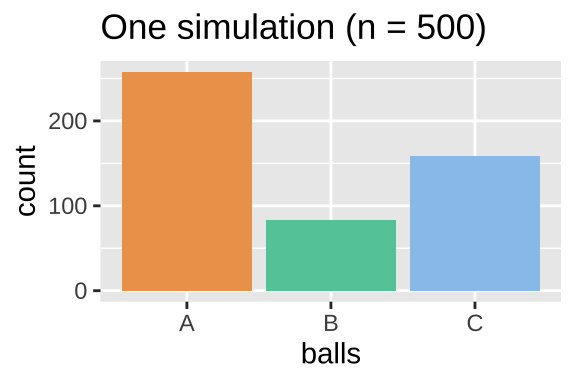#### Simulations to find the proportions of OR and NOT

Say we wanted to count the number of balls that fell through A, B.

Remember that the proportion of a sample that is $$a$$ OR $$b$$, (aka $$Pr_(a \text{ or } b)$$ is the proportion that are $$a$$ ($$Pr_a$$) plus the proportion that are $$b$$ ($$Pr_b$$) minus the probability of $$A$$ and $$B$$, $$Pr(AB)$$ – which equals zero when events are mutually exclusive. In R we find this as

ball_dist_exclusive %>%
summarise(Pr_A = mean(balls == "A"),
Pr_B = mean(balls == "B"),
Pr_AorB = Pr_A + Pr_B)
## # A tibble: 1 × 3
##    Pr_A  Pr_B Pr_AorB
##   <dbl> <dbl>   <dbl>
## 1 0.532  0.15   0.682

Alternatively, we could simply use the OR | operator

ball_dist_exclusive %>%
summarise(Pr_AorB = mean(balls == "A" | balls == "B"))
## # A tibble: 1 × 1
##   Pr_AorB
##     <dbl>
## 1   0.682

Because A, B and C make up all of sample space, we could find the proportion A or B as one minus the proportion of C.

ball_dist_exclusive %>%
dplyr::summarise(p_AorB = 1- mean(balls == "C") )
## # A tibble: 1 × 1
##   p_AorB
##    <dbl>
## 1  0.682

Note that all these ways of finding $$Pr(A\text{ or }B)$$ are all equivalent, and are close to our expected value of $$1/2+1/6 = 0.667$$

## 15.3Simulating proportions for many samples in R

We can also use the sample() function to simulate a sampling distribution. So without any math tricks.

There are a bunch of ways to do this but my favorite recipe is:

1. Make a sample of size sample_size $$\times$$ n_reps.
2. Assign the first sample_size outcomes to the first replicate, the second sample_size outcomes to the second replicate etc…
3. Summarize the output.

Here I show these steps

1. Make a sample of size sample_size $$\times$$ n_reps.

n_reps   <- 1000
ball_sampling_dist_exclusive <- tibble(balls = sample(x = c("A","B","C"),
size  = n_balls * n_reps, # sample 500 balls 1000 times
replace = TRUE,
prob = c(P_A, P_B, P_C))) 

2. Assign the first sample_size outcomes to the first replicate, the second sample_size outcomes to the second replicate etc…

ball_sampling_dist_exclusive <- ball_sampling_dist_exclusive %>%
mutate(replicate = rep(1:n_reps, each = n_balls))

Let’s have a look at the result looking at only the first 2 replicates:

3. Summarize the output.

ball_sampling_dist_exclusive <- ball_sampling_dist_exclusive %>%
group_by(replicate, balls, .drop=FALSE) %>% # make sure to keep zeros
dplyr::summarise(count = n(),.groups = "drop")  # count number of balls of each color each replicate

Let’s have a look at the result

MAKE A PLOT

ggplot(ball_sampling_dist_exclusive, aes(x = count, fill = balls)) +
geom_density(alpha = .8, show.legend = FALSE, adjust =1.5)+
scale_fill_manual(values = ball_colors)+
geom_vline(xintercept =  n_balls * c(P_A, P_B, P_C), lty = 2,  color = ball_colors)+
annotate(x = n_balls * c(P_A, P_B, P_C), y = c(.02,.02,.02),
geom = "text",label = c("A","B","C"), size = 6 )+
labs(title = "Many simulations")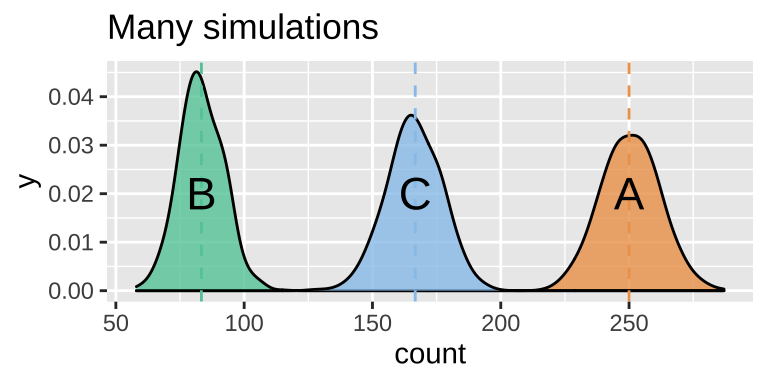Figure 15.1: Sampling distribution of the number of outcomes A, B and C.

SUMMARIZE UNCERTAINTY

We can estimate the variability in a random estimate from this population with a sample of size 500 (the standard error) as the standard deviation of the density plots, in Fig. 15.1.

We do so as follows:

ball_sampling_dist_exclusive %>%
group_by(balls) %>%
dplyr::summarise(se = sd(count))
## # A tibble: 3 × 2
##   balls    se
##   <chr> <dbl>
## 1 A     11.0
## 2 B      8.61
## 3 C     10.5

## 15.4 Simulating Non-Exclusive events

We just examined a case in which a ball could not fall through both A and B, so all options where mutually exclusive.

Recall that this need not be true. Let us revisit a case when falling through A and B are not exclusive.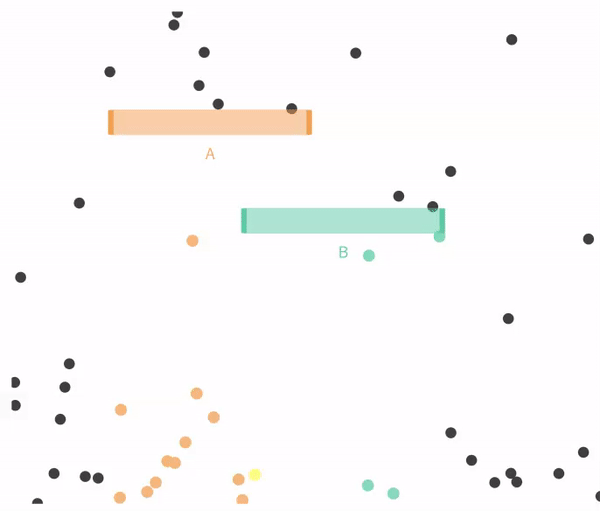### 15.4.1 Simulating independent events

Recal that events are independent if the the occurrence of one event provides no information about the other. Let us consider the case of in which $$A$$ and $$B$$ are independent (below).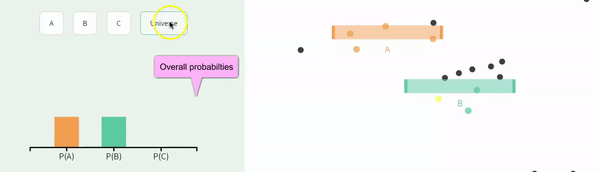We can simulate independent events as two different columns in a tibble, as follows.

p_A <- 1/3
p_B <- 1/3
nonexclusive1 <- tibble(A = sample(c("A","not A"),
size = n_balls,
replace = TRUE,
prob = c(p_A, 1 - p_A)),
B = sample(c("B","not B"),
size = n_balls,
replace = TRUE,
prob = c(p_B, 1 - p_B)))

Summary counts

nonexclusive1 %>%
group_by(A,B)%>%
dplyr::summarise(count = n(), .groups = "drop")  %>%# lose all groupings after sumarising
mutate(expected_count = 500 * c(1/3 * 1/3, 2/3 * 1/3, 1/3 * 2/3, 2/3 * 2/3),
observed_prop  = count / sum(count),
expected_prop  = expected_count /500
)
## # A tibble: 4 × 6
##   A     B     count expected_count observed_prop expected_prop
##   <chr> <chr> <int>          <dbl>         <dbl>         <dbl>
## 1 A     B        58           55.6         0.116         0.111
## 2 A     not B   113          111.          0.226         0.222
## 3 not A B       112          111.          0.224         0.222
## 4 not A not B   217          222.          0.434         0.444

A plot

ggplot(nonexclusive1, aes(x =A, fill = B))+
geom_bar(position = "dodge")+
scale_fill_manual(values = c("black","grey"))+
theme_light()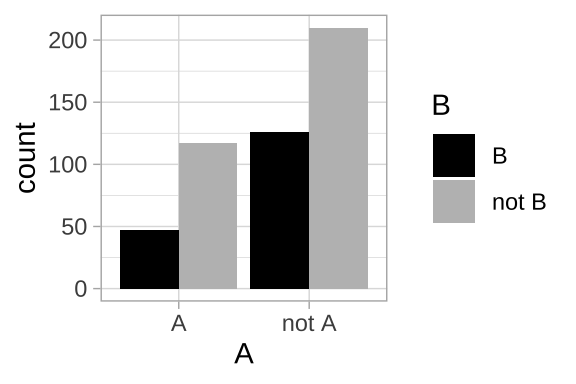Remember that the proportion of a sample that is $$a$$ OR $$b$$, (aka $$Pr_(a \text{ or } b)$$ is the proportion that are $$a$$ ($$Pr_a$$) plus the proportion that are $$b$$ ($$Pr_b$$) minus the probability of $$A$$ and $$B$$, $$Pr(AB)$$ – which does not equal zero for non-exclusive events. Let’s make sure this works with some light R coding!

nonexclusive1 %>%
summarise(Pr_A  = mean(A == "A"),
Pr_B  = mean(B == "B"),
Pr_AorB =  mean(A == "A" | B == "B"),
# lets verify the general addition principle by
# summing and subtracting double counts
Pr_AandB = mean(A == "A" & B == "B"),
Pr_AorBmath = Pr_A + Pr_B - Pr_AandB
)
## # A tibble: 1 × 5
##    Pr_A  Pr_B Pr_AorB Pr_AandB Pr_AorBmath
##   <dbl> <dbl>   <dbl>    <dbl>       <dbl>
## 1 0.342  0.34   0.566    0.116       0.566

### 15.4.2 Simulating Non-independece

Recall that events are non-independent if their conditional probabilities differ from their unconditional probabilities. Let us revisit our example of non-independence from the previous chapter:

• The probability of A, P(A) = $$\frac{1}{3}$$.

• The probability of A conditional on B, P(A|B) = $$\frac{1}{4}$$.

• The probability of B, P(B) = $$\frac{2}{3}$$.

• The probability of B conditional on A, P(B|A) = $$\frac{1}{2}$$.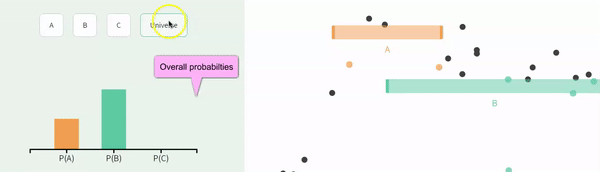Figure 15.2: Non-independence

So, how to simulate conditional probabilities? The easiest way to do this is to:

• Simulate one variable first.
• Simulate another variable next, with appropriate conditional probabilities.

So let’s do this, simulating A first

p_A <- 1/3
nonindep_sim <- tibble(A = sample(c("A","not A"), size = n_balls,
replace = TRUE, prob = c(p_A, 1 - p_A)))

Now we simulate $$B$$. But first we need to know

• P(B|A) which we know to be $$\frac{1}{2}$$, and
• P(B| not A), which we don’t know. We’ll see hw we could calculate that value in the next chapter. For now, I will tell you that p(B| not A) = 3/4.
We also use one new R trick – the case_when() function. The fomr of this is

case_when(<thing meets some criteria> ~ <output something>, <thing meets different criteria> ~ <output somthing else>)

We can have as many cases as we want there! This is a lot like the ifelse() function that you may have seen elsewhere, but is easier and safer to use.

p_B_given_A    <- 1/2
p_B_given_notA <- 3/4
nonindep_sim   <- nonindep_sim %>%
group_by(A) %>%
mutate(B = case_when(A == "A" ~ sample(c("B","not B"), size = n(),
replace = TRUE, prob = c(p_B_given_A  , 1  - p_B_given_A )),
A != "A" ~ sample(c("B","not B"), size = n(),
replace = TRUE, prob = c(p_B_given_notA  , 1  - p_B_given_notA))
)) %>%
ungroup()

Let’s see what we got!!

#### Making sure simulations worked

It is always worth doing some checks to make sure our simulation isn’t too off base – let’s make sure that

about 2/3 of all balls went through B (taking the mean of a logical to find the proportion).

nonindep_sim %>%
summarise(total_prop_B = mean(B=="B")) 
## # A tibble: 1 × 1
##   total_prop_B
##          <dbl>
## 1        0.638

about 1/2 of As went through B and 3/4 of not A went through B.

nonindep_sim %>%
group_by(A) %>%
summarise(conditional_prop_B = mean(B=="B")) 
## # A tibble: 2 × 2
##   A     conditional_prop_B
##   <chr>              <dbl>
## 1 A                  0.430
## 2 not A              0.734
Complex simulations can be tough, so I always recommend quick spot checks like those above.
When doing these checks, remember that sampling error is smallest with large sample sizes, so trying something out with a large sample size will help you better see signal.

#### 15.4.2.1 Recovering Bayes’ theorem from our simulation results

In the previous chapter we introduced Bayes’ theorem $$P(A|B) = \frac{P(B|A) P(A)}{P(B)}$$ allows us to flip conditional probabilities. I think its easier to understand this as a simple proportion. Consider

• $$Pr(A|B)$$ as the proportion of outcomes A out of all cases when we see B,

• $$Pr(B|A) \times Pr(A)$$ as the proportion of times we observe B and A.

• $$Pr(B)$$ as the proportion of times we observe B.

That is, the proportion of times we get A when we have B is simply the number of times we see A and B divided by the number of times we see A.

So we can find the proportion of balls that

• Fell through A and B,
• Fell through neither A nor B,
• Fell through A not B,
• Fell through B not A,
nonindep_sim %>%
group_by(A, B, .drop = FALSE) %>%
summarise(prop = n() / n_balls)
## summarise() has grouped output by 'A'. You can override using the .groups argument.
## # A tibble: 4 × 3
## # Groups:   A 
##   A     B      prop
##   <chr> <chr> <dbl>
## 1 A     B     0.136
## 2 A     not B 0.18
## 3 not A B     0.502
## 4 not A not B 0.182

We can also find, for example, the proportion of balls that

• Fell through A conditional on not falling through B.
nonindep_sim %>%
filter(B == "not B")  %>%
summarise(prop_A_given_notB = mean(A == "A"))
## # A tibble: 1 × 1
##   prop_A_given_notB
##               <dbl>
## 1             0.497

This value of approximately 1/2 lines up with a visual estimate that about half of the space not taken up by B in Figure 14.5 is covered by A.

Tips for conditional proportions.
To learn the proportion of events with outcome a given outcome b, we divide the proportion of outcomes with both a and b by the proportion of outcomes all outcomes with a and b by the proportion of outcomes with outcome b. $prop(a|b) = \frac{prop(a\text{ and }b)}{prop(b)}$

This is the basis for Bayes theorem.

## 15.5 A biologically inspired simulation

Let us revisit our flu vaccine example to think more biologically about Bayes’ theorem. First, we lay out some rough probabilities:

• Every year, a little more than half of the population gets a flu vaccine, let us say P(No Vaccine) = 0.58.

• About 30% of people who do not get the vaccine get the flu, P(Flu|No Vaccine) = 0.30.

• The probability of getting the flu diminishes by about one-fourth among the vaccinated, P(Flu|Vaccine) = 0.075.

So you are way less likely to get the flu if you get the flu vaccine, but what is the probability that a random person who had the flu vaccine P(Vaccine|Flu)? We grinded through the mast last chapter - let’s see if simulation helps us think. To do so we simulate from these conditional probabilities, count, and divid the number of people that had the flu and the vaccine by the number of people who had the flu.

p_Vaccine             <- 0.42
p_Flu_given_Vaccine   <- 0.075
p_Flu_given_NoVaccine <- 0.300
n_inds                <- 10000000

flu_sim <- tibble(vaccine = sample(c("Vaccinated","Not Vaccinated"),
prob =c(p_Vaccine, 1- p_Vaccine),
size = n_inds,
replace = TRUE )) %>%
group_by(vaccine) %>%
mutate(flu = case_when(vaccine == "Vaccinated" ~ sample(c("Flu","No Flu"),
size = n(),
replace = TRUE,
prob = c(p_Flu_given_Vaccine, 1  - p_Flu_given_Vaccine)),
vaccine != "Vaccinated" ~ sample(c("Flu","No Flu"),
size = n(),
replace = TRUE,
prob = c(p_Flu_given_NoVaccine, 1  - p_Flu_given_NoVaccine))
)) %>%
ungroup()

Let’s browse the first 1000 values

Let’s find all the proportion of each combo

flu_sum <- flu_sim %>%
group_by(vaccine, flu, .drop = FALSE) %>%
summarise(sim_prop = n() / n_inds, .groups = "drop") 

Compare to predictions

Recal that we fund predicitons from math:

$\begin{equation} \begin{split} P(\text{Vaccine|Flu}) &= P(\text{Vaccine and Flu}) / P(\text{Flu}) \\ P(\text{Vaccine|Flu}) &= \tfrac{P(\text{Flu|Vaccine}) \times P(\text{Vaccine})}{P(\text{Vaccine}) \times P(\text{Flu}|\text{Vaccine}) + P(\text{No Vaccine}) \times P(\text{Flu}|\text{No Vaccine})}\\ P(\text{Vaccine|Flu}) &= 0.0315 / 0.2055 \\ P(\text{Vaccine|Flu}) &=0.1533 \end{split} \end{equation}$

So, while the vaccinated make up 42% of the population, they only make up 15% of people who got the flu.

precitions <- tibble(vaccine   = c("Not Vaccinated", "Not Vaccinated", "Vaccinated", "Vaccinated"),
flu       = c("Flu", "No Flu", "Flu", "No Flu"),
math_prob = c((1-p_Vaccine) * (p_Flu_given_NoVaccine) ,
(1-p_Vaccine) * (1- p_Flu_given_NoVaccine),
(p_Vaccine) * (p_Flu_given_Vaccine),
(p_Vaccine) * (1-p_Flu_given_Vaccine)))

full_join(flu_sum, precitions)       
## # A tibble: 4 × 4
##   vaccine        flu    sim_prop math_prob
##   <chr>          <chr>     <dbl>     <dbl>
## 1 Not Vaccinated Flu      0.174     0.174
## 2 Not Vaccinated No Flu   0.406     0.406
## 3 Vaccinated     Flu      0.0316    0.0315
## 4 Vaccinated     No Flu   0.388     0.388

Now let’s check our flipping of conditional probabilities (aka Bayes’ theorem)

flu_sum %>%
filter(flu == "Flu") %>% # only looking at people with flu
mutate(prop_given_flu = sim_prop / sum(sim_prop))
## # A tibble: 2 × 4
##   vaccine        flu   sim_prop prop_given_flu
##   <chr>          <chr>    <dbl>          <dbl>
## 1 Not Vaccinated Flu     0.174           0.846
## 2 Vaccinated     Flu     0.0316          0.154

This is basically what we got by math (recall $$P(\text{Vaccine|Flu}) =0.1533$$ from the previous chapter).

## 15.6 How to do probability – Math or simulation?

In the previous chapter we saw that we could work through a bunch of proababilistic thinking mathematically. In fact – everything we cover here could be discovered by math. This holds not only for these chapters, but for pretty much all of stats.

But for me, simulation provides us with a good intuition, every problem we cover this term could be exactly solved by simulation (while math requires numerous assumptions), and some situations can uniquely solve problems that do not have nice mathematical answers. I often wonder – “Why do we even use math?”

The major advantages of math over simulation are:• Math gives the same answers every time. Unlike random simulation, math is not influenced by chance, so (for example) a sampling error on our computer mean that the sampling distribution from simulation will differ slightly each time, while math based sampling distributions do not change by chance.

• Math is fast. Simulations can take a bunch of computer time and energy (it has to do the same thing a bunch of times). With math, the computer quickly run some numbers through a calculator. The computational burdens we’ll face this term are pretty minimal, but with more complex stats this can actually matter.

• Math provides insight. Simulations are great for helping us build feelings, intuition and even probabilities. But clear mathematical equations allow us to precisely see the relationship between components of our model.

## 15.7 Probabilistic simulations: Quiz

Go through all “Topics” in the learnR tutorial, below. Nearly identical will be homework on canvas.

### References

Humphrey, Parris T, and Joanna Masel. 2016. “Outcome Orientation: A Misconception of Probability That Harms Medical Research and Practice.” Perspectives in Biology and Medicine 59 (2): 147–55.
Whitlock, Michael C, and Dolph Schluter. 2020. The Analysis of Biological Data. Third Edition.